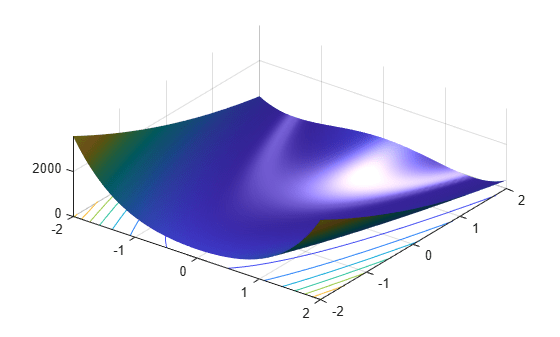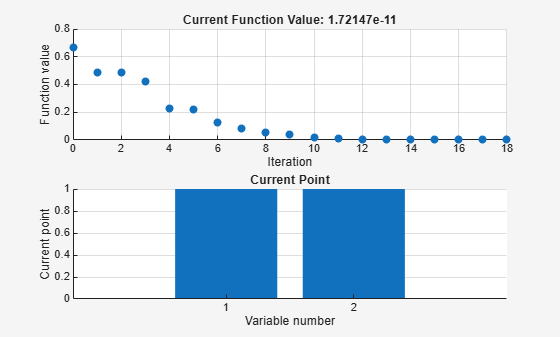# Hybrid Scheme in the Genetic Algorithm

This example shows how to use a hybrid scheme to optimize a function using the genetic algorithm and another optimization method. `ga` can quickly reach a neighborhood of a local minimum, but it can require many function evaluations to achieve convergence. To speed the solution process, first run `ga` for a small number of generations to approach an optimum point. Then use the solution from `ga` as the initial point for another optimization solver to perform a faster and more efficient local search.

### Rosenbrock's Function

This example uses Rosenbrock's function (also known as Dejong's second function) as the fitness function:

$f\left(x\right)=100\left(x\left(2\right)-x\left(1{\right)}^{2}{\right)}^{2}+\left(1-x\left(1\right){\right)}^{2}$.

Rosenbrock's function is notorious in optimization because of the slow convergence most methods exhibit when trying to minimize this function. Rosenbrock's function has a unique minimum at the point x* = (1,1), where it has a function value $f\left({x}^{*}\right)=0$.

The code for Rosenbrock's function is in the `dejong2fcn` file.

`type dejong2fcn.m`
```function scores = dejong2fcn(pop) %DEJONG2FCN Compute DeJongs second function. %This function is also known as Rosenbrock's function % Copyright 2003-2004 The MathWorks, Inc. scores = zeros(size(pop,1),1); for i = 1:size(pop,1) p = pop(i,:); scores(i) = 100 * (p(1)^2 - p(2)) ^2 + (1 - p(1))^2; end ```

Plot Rosenbrock's function over the range –2 <= x(1) <= 2; –2 <= x(2) <=2.

`plotobjective(@dejong2fcn,[-2 2;-2 2]);`### Genetic Algorithm Solution

First, use `ga` alone to find the minimum of Rosenbrock's function.

```FitnessFcn = @dejong2fcn; numberOfVariables = 2;```

Include plot functions to monitor the optimization process.

`options = optimoptions(@ga,'PlotFcn',{@gaplotbestf,@gaplotstopping});`

Set the random number stream for reproducibility, and run `ga` using the options.

```rng default [x,fval] = ga(FitnessFcn,numberOfVariables,[],[],[],[],[],[],[],options)```
```Optimization terminated: average change in the fitness value less than options.FunctionTolerance. ``````x = 1×2 0.3454 0.1444 ```
```fval = 0.4913 ```

Using the default stopping criteria, `ga` does not provide a very accurate solution. You can change the stopping criteria to try to find a more accurate solution, but `ga` requires many function evaluations to approach the global optimum x* = (1,1).

Instead, perform a more efficient local search that starts where `ga` stops by using the hybrid function option in `ga`.

A hybrid function begins from the point where `ga` stops. Hybrid function choices are `fminsearch`, `patternsearch`, or `fminunc`. Because this optimization example is smooth and unconstrained, use `fminunc` as the hybrid function. Provide `fminunc` with plot options as an additional argument when specifying the hybrid function.

```fminuncOptions = optimoptions(@fminunc,'PlotFcn',{'optimplotfval','optimplotx'}); options = optimoptions(options,'HybridFcn',{@fminunc, fminuncOptions});```

Run `ga` again with `fminunc` as the hybrid function.

`[x,fval] = ga(FitnessFcn,numberOfVariables,[],[],[],[],[],[],[],options)`
```Optimization terminated: average change in the fitness value less than options.FunctionTolerance. ``````Local minimum found. Optimization completed because the size of the gradient is less than the value of the optimality tolerance. ``````x = 1×2 1.0000 1.0000 ```
```fval = 1.7215e-11 ```

The first plot shows the best and mean values of the population in every generation. The plot title identifies the best value found by `ga` when it stops. The hybrid function `fminunc` starts from the best point found by `ga`. The second plot shows the solution `x` and `fval`, which result from using `ga` and `fminunc` together. In this case, using a hybrid function improves the accuracy and efficiency of the solution.

Watch now